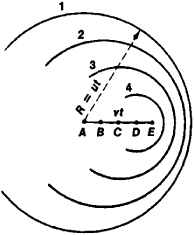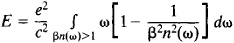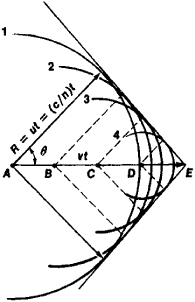Also found in: Dictionary, Medical, Wikipedia.

or

[for P. A. CherenkovCherenkov, Pavel Alekseyevich
, 1904–90, Soviet physicist. He shared with the Soviet physicists I. M. Frank and I. Y. Tamm the 1958 Nobel Prize in Physics for his discovery (1934) of Cherenkov radiation.
], light emitted by a transparent medium when charged particles pass through it at a speed greater than the speed of light in the medium. The effect, discovered by Cherenkov in 1934 while he was studying the effects of gamma rays on liquids and explained in 1937 by I. E. TammTamm, Igor Yevgenyevich,
1895–1971, Soviet physicist, Ph.D. Moscow State Univ., 1933. He was a researcher at the P. N. Lebedev Physical Institute in Moscow from 1934 until his death in 1971. Tamm and Ilya M.
and I. M. FrankFrank, Ilya Mikhailovich,
1908–90, Soviet physicist, Ph.D. Moscow State Univ., 1935. He was a professor at Moscow State Univ. from 1944 until his death in 1990. Mikhailovich and Igor Y. Tamm won the 1958 Nobel Prize in Physics with Pavel A.
, is analogous to the creation of a sonic boomsonic boom,
shock wave produced by an object moving through the air at supersonic speed, i.e., faster than the speed of sound. Since sound is a mechanical disturbance that propagates through the air, there is a limit to its speed.
when an object exceeds the speed of sound in a medium. The light is emitted only in directions inclined at a certain angle to the direction of the particles' motion dependent upon the particles' momentum. Thus, by simply measuring the angle between the radiation and the path of the particles, the particles' speed may be determined. The effect is used in the Cherenkov counter, a device for detecting fast particles and determining their speeds or distinguishing between particles of different speeds.

(also Čerenkov radiation, Cherenkov effect, or Čerenkov effect), the emission of light by a charged particle when the particle moves in a medium at a velocity greater than the phase velocity of light waves in the medium, that is, greater than the velocity at which light waves propagate in the medium. Cherenkov radiation was discovered in 1934 by P. A. Cherenkov when, during a study of the gamma-ray luminescence of solutions, he observed a faint blue glow in liquids irradiated by gamma rays.

Even the early experiments of Cherenkov, which were undertaken on the initiative of S. I. Vavilov, revealed a number of characteristic features of Cherenkov radiation. The early experiments showed that the glow is observed in all pure transparent liquids, the brightness of the glow is weakly dependent on the chemical composition of such a liquid, and the radiation is polarized with the electric vector preferentially oriented parallel to the direction in which the charged-particle beam propagates. In contrast to luminescence, neither temperature quenching nor impurity quenching (seeQUENCHING OF LUMINESCENCE) of the glow was observed in the experiments. On the basis of the observational data, Vavilov contended that the phenomenon discovered by Cherenkov was not the luminescence of a liquid and that the light was emitted by high-speed electrons moving in the liquid; during gamma irradiation, such electrons arise as a result of the Comp-ton effect. Therefore, the phenomenon would more correctly be referred to as Vavilov-Cherenkov radiation, or the Vavilov-Cherenkov effect, rather than as the Cherenkov effect, which is the conventional term used, especially in the non-Soviet literature. Cherenkov radiation is also characteristic of solids.

Different types of glows caused by gamma rays were repeatedly observed after the discovery of radium. In particular, the glow in liquids irradiated by gamma rays was investigated between 1926 and 1929 by the French scientist M. L. Mallet, who obtained photographs of the glow’s spectrum. However, no evidence that the glow was a new phenomenon was obtained, and the most characteristic property of the radiation—namely, its directivity at an acute angle to the direction of motion of the particle—was not established. The directivity of the radiation was discovered by Cherenkov in 1936.

The mechanism of the Cherenkov effect was explained in a paper published by I. E. Tamm and I. M. Frank in 1937. The paper also contained a quantitative theory based on the equations of classical electrodynamics. A quantum treatment by V. L. Ginzburg in 1940 led to the same results.

The conditions under which Cherenkov radiation occurs and the directivity of the radiation may be explained by means of the Huygens-Fresnel principle. For this purpose, each point of the trajectory of a charged particle—for example, the point A, B, C, or D in Figures 1 and 2—should be regarded as the source of a secondary wave, which is generated when the charge passes through that point. In an optically isotropic medium, such secondary waves are spherical since they propagate in all directions at the same velocity u = c/n, where c is the speed of light in vacuum and n is the refractive index of a given medium. Let us assume that a particle moving with velocity v is at point E at the moment of observation. Since the distance between points A and E is equal to vt, t seconds before the particle is observed at E, it passes through A. Consequently, by the moment of observation, the wave emanating from A is a sphere of radius R = ut (the circle 1 in Figures 1 and 2 corresponds to the sphere). Light is emitted from B, C, and D at increasingly later moments in time; the waves emanating from these points are represented by the circles 2, 3, and 4, respectively. According to Huygens’ principle, as a result of interference, secondary waves cancel one another everywhere except at their common envelope. The wavefront of the light propagating in the medium corresponds to the common envelope.

Let the particle velocity v be less than the speed of light u in a medium (Figure 1). Then, the earlier the emission of forward-propagating light, the greater the distance at which the light overtakes the particle. In this case, the secondary waves do not have a common envelope; that is, all the circles 1, 2, 3, and 4 lie inside one another. This situation corresponds to the obvious fact that an electric charge undergoing uniform rectilinear motion at a speed less than the speed of light in a medium should not emit light.Figure 1. The motion of a charged particle in a medium at a velocity of v < u: the circles 1, 2, 3, and 4 represent the locations of the secondary waves emitted by the particle from points A, B, C, and D, respectively

However, the situation is different if

(1) v > u = c/n, or βn > 1

where β = v/c, that is, if the particle moves faster than the light waves. In this case, the spheres corresponding to the light waves intersect (Figure 2). The common envelope of the light waves, or the wavefront, is a cone with its vertex at E, which coincides with the instantaneous position of the particle. The normals to the generators of the cone give the wave vectors, that is, the direction in which the light propagates. The angle that a wave vector makes with the direction of the particle’s motion (see Figure 2) satisfies the relation

(2) cos θ = u/v = c/nv = 1/βn

The same method may be used to consider optically anisotropic media. However, the fact that the speed of light in such a medium depends on the direction in which the light propagates must be taken into account. Therefore, the secondary waves are not spheres. In this case, different cones correspond to ordinary and extraordinary rays and, in accordance with equation (2), the radiation is emitted at various angles θ to the direction in which the particle propagates. For optically anisotropic media, criterion (1) is formulated somewhat differently. In all cases, the basic equations of the theory are in good agreeent with experiments.

Theory has shown that, in an optically isotropic medium, a particle of charge e traveling a distance of 1 cm at a velocity of v > u radiates an energy ofwhere ω = 2nc/λ is the circular frequency of the light; here, λ isFigure 2. The motion of a charged particle in a medium at a velocity of v > u: the angle θ indicates the direction of the emitted radiation

the vacuum wavelength of the emitted light. The integrand gives the spectral energy distribution of the Cherenkov radiation, while the bounds of the domain of integration are specified by criterion (1).

Cherenkov radiation is produced not only when an electron moves in a medium but when any charged particle moves in a medium, provided criterion (1) is satisfied for the particle. For electrons in liquids and solids, criterion (1) is satisfied at energies of as low as ~105 electron volts (eV); many electrons associated with radioactive processes have such energies. Heavier particles should have higher energies. For example, to attain the required velocity, a proton, whose mass is ~2,000 times greater than the mass of an electron, should have an energy of ~108 eV. Such protons may be produced only in present-day accelerators.

Cherenkov radiation has been used to develop experimental methods that are widely employed in nuclear physics both to detect particles and to study the nature of particles (seeCHERENKOV COUNTER). The velocity of a particle may be obtained from equation (2) or from criterion (1) by measuring θ in a medium or transparent substance for which n is known or by determining the radiation threshold. After a particle’s velocity is obtained and its energy is determined on the basis of its deflection in a magnetic field, the mass of the particle may be calculated. Such a procedure was used, for example, in the discovery of the antiproton. For ultrarelativistic particles, criterion (1) is satisfied in compressed gases, such as those used in gas Cherenkov counters. The Cherenkov radiation that is produced in the earth’s atmosphere is used to study cosmic rays.

Cherenkov radiation may be observed in pure form only in ideal cases, where a particle moves with constant velocity in a transparent substance of unlimited length. When a particle traverses the surface of a transparent substance, transition radiation is produced. Such radiation was predicted theoretically by Ginzburg and Frank in 1946 and was later investigated experimentally. The essence of transition radiation consists in the fact that the electromagnetic field of a particle is different in a vacuum and in a medium. Any change in the particle’s field always results in the emission of light. For example, in the case of bremsstrahlung, the emission is caused by a change in the particle’s velocity; in the case of transition radiation, the emission results from a change in the electromagnetic properties of the medium along the particle’s trajectory. In a thin transparent substance that satisfies criterion (1), transition radiation is indistinguishable from Cherenkov radiation to a certain extent. At the surface of an opaque substance, the emitted transition radiation is more in evidence since the intensity of the Cherenkov radiation is reduced by absorption.

Transition radiation is also produced when criterion (1) is not satisfied—for example, at low particle velocities or, in contrast, when an ultrarelativistic particle radiates in the X-ray region, where n < 1 and, consequently, βn is always less than unity. The intensity of transition radiation is low and is usually inadequate for the detection of an individual particle. For the effective detection of transition radiation, the radiation emitted by a particle as it traverses several interfaces in succession may be summed.

In 1940, E. Fermi, taking into account the ability of a real medium to absorb light in at least several spectral regions, generalized the theory of Cherenkov radiation. The results obtained by Fermi substantially improved the theory of ionization losses by charged particles, which deals with the effect of the polarization of a medium.

Cherenkov radiation is an example of “faster-than-light” optics and is of fundamental importance. It is studied experimentally and theoretically not only in optically isotropic media but also in crystals, which are optically anisotropic media. Electric and magnetic dipole and multipole radiation is considered theoretically. The expected properties of the radiation of a moving magnetic charge have been used to search for magnetic mono-poles. The radiation of a particle in a channel within a medium—for example, the radiation of a particle beam in a wave guide—has been examined. When Cherenkov radiation is emitted, the Doppler effect in a medium takes on new characteristics, as shown by the occurrence of anomalous and complex Doppler effects. It may be assumed that any system of particles that is capable of interacting with an electromagnetic field will emit light owing to its own kinetic energy if the velocity of the system exceeds the phase velocity of light waves.

The theoretical concepts that underlie Cherenkov radiation are closely associated with other phenomena of importance in contemporary physics, such as Mach waves in acoustics, some aspects of the stability of particle motion in a plasma and of wave generation in a plasma, certain problems in the theory of particle accelerators, and the generation and amplification of electromagnetic waves.

### REFERENCES

Cherenkov, P. A. “Vidimoe svechenie chistykh zhidkostei pod deistviem γ-radiatsii.” Dokl. AN ASSR, 1934, vol. 2, no. 8.
Vavilov, S. I. “O vozmozhnykh prichinakh sinego γ-svecheniia zhidkostei.” Ibid.
Tamm, I. E., and I. M. Frank. “Kogerentnoe izluchenie bystrogo elektrona v srede.” Dokl. AN ASSR, 1937, vol. 14, no. 3.
Cherenkov, P. A., I. E. Tamm, and I. M. Frank. Nobelevskie lektsii. Moscow, 1960.
Jelley, J. Cherenkovskoe izluchenie i ego primeneniia. Moscow, 1960. (Translated from English.)
Zrelov, V. P. Izluchenie Vavilova-Cherenkova i ego primenenie v fizike vysokikh energii, parts 1–2. Moscow, 1968.

I. M. FRANK

References in periodicals archive ?
The Cherenkov radiation is emitted in the form of dispersive wave at a frequency underlying phase matching condition.
From the angle at which Cherenkov radiation is emitted, the speed of ultrafast particles can be calculated, and for this finding, Cherenkov, Tamm, and Frank were awarded the Nobel Prize for physics in 1958.
In addition to thermal radiation and Cherenkov radiation, Van Dover and her colleagues have thought of several other mechanisms that might be producing the light.
1 we observe that for frequencies [[omega].sub.0] [less than or equal to] 3 the angle [[theta].sub.1] is negative what corresponds to the reverse Cherenkov radiation. But for larger [[omega].sub.0] [greater than or equal to] 6 the Cherenkov radiation already acquires conventional structure with positive [[theta].sub.1] > 0, see Fig.
Their mostly blue light is known as Cherenkov radiation, after the Russian physicist who first studied the effect in the mid-'30s.
The muon is electrically charged (the neutrino is not) and emits the kind of light called Cherenkov radiation as it moves through the water.
Cherenkov radiation by a charged source that moves in (or in the interface) a left-handed material has been studied in number of works [15-21].
Rather, it sees Cherenkov radiation from relativistic muons, which are essentially superheavy electrons that are spawned when an unlucky neutrino interacts with an atom.
Kamiokande II contains 2,000 tons of water, in which neutrinos can strike either electrons or free protons to produce Cherenkov radiation, a kind of light produced when a particle moves at a speed greater than the speed of light in water.

Site: Follow: Share:
Open / Close